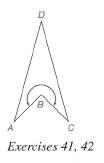Chapter 4.2, Problem 41EElementary Geometry For College St...

7th Edition
Alexander + 2 others
ISBN: 9781337614085

Solutions

Chapter
SectionElementary Geometry For College St...

7th Edition
Alexander + 2 others
ISBN: 9781337614085
Textbook Problem

In concave kite A B C D , there is an interior angle at vertex B that is a reflex angle. Given that m ∠ A = m ∠ C = m ∠ D = 30 ° , find the measure of the indicated reflex angle.To determine

To find:

The measure of the indicated reflex angle.

Explanation

Calculation:

Given,

A concave kite ABCD

mA=mC=mD=30°

In a kite the sum of all the interior angles is 360°.

Thus,

A+B+C+D=360°

Still sussing out bartleby?

Check out a sample textbook solution.

See a sample solution

The Solution to Your Study Problems

Bartleby provides explanations to thousands of textbook problems written by our experts, many with advanced degrees!

Get Started

Evaluate the integral. 01(1+r)3dr

Calculus: Early Transcendentals

In Exercises 1-6, simplify the expression. 5(1)26(1)+9

Calculus: An Applied Approach (MindTap Course List)

13. Write the formula for the housing expense ratio. (14-7)

Contemporary Mathematics for Business & Consumers

The radius of convergence of is: 1 3 ∞

Study Guide for Stewart's Single Variable Calculus: Early Transcendentals, 8th

In Exercise 13-22, perform the indicated operations. 23

Finite Mathematics for the Managerial, Life, and Social Sciences